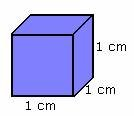## Definition Of Cubic Unit

Volume of the solids is measured in Cubic Units.

A milliliter is equivalent to one cubic centimeter.
One liter is equivalent to one cubic decimeter.
One kiloliter is equivalent to one cubic meter.

### Example of Cubic UnitThe given figure is a cube. The length of each side of the cube is 1 centimeter, so its volume is 1 cubic centimeter.

### Solved Example on Cubic Unit

#### Ques: Identify a solid that has a volume of 32 cubic units.A. Figure 1
B. Figure 2
C. Figure 3
D. Figure 4